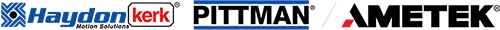# What do brushless motor's winding parameters mean and how to choose?

When designing a Brushless Motor into your application there is more to consider than just the torque rating. Choosing a proper winding is crucial for proper operation.Figure 1: To determine suitable motor winding

The motor equation 1, (Figure 1) helps in determining which winding is suitable for your available power source. This is where the ‘other’ winding parameters come into play.

Where:= Required voltage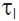= Torque of the load (max torque of profile. Usually acceleration torque)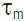= Friction torque and damping loss of the motor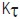= Torque Constant= line to line resistance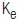= Voltage constant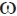= Motor speed
L di/dt = Additional voltage required for the switch inductanceVoltage Constant
Voltage constant can also be called back-EMF constant. When the rotor rotates, it generates a voltage proportional to speed. This voltage opposes the applied voltage. When the generated voltage approaches the value of applied voltage, the motor reaches its final speed. Hence, applied voltage is proportional to motor speed. The back-EMF constant is expressed in V/KRPM or volts/rad/sec. In the equation below (Figure 2), the product of the voltage constant (volts/rad/sec) and motor speed (rad/sec) will equal the required voltage for the desired motor speed. Note that the units are extremely important.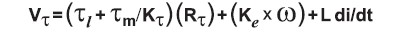Figure 2: Voltage constant in a motor winding equation.Torque Constant
The torque constant is the proportional relationship between current and torque. Usually expressed in torque per amps such as oz-in/amp or Nm/amp. In the equation below (Figure 3), the ‘total’ torque (load and frictional) divided by the torque constant will give you the required current, which multiplied by the resistance will give you the voltage drop across the winding.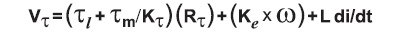Figure 3: Torque constant in a motor winding equation.Resistance
The DC resistance (Figure 4) is the line to line resistance of the stator expressed in ohms. The value listed in the data sheet is usually the value at room temperature (22°C). When used in the motor equation, it is advised to use the ‘hot’ resistance. This is the value of the resistance at maximum winding temperature.Figure 4: DC resistance constant in a motor winding equationInductance
When commutating a brushless motor, each step is energizing
and de-energizing a phase. The ‘switching inductance’ requires additional voltage. Ldi/dt defines this voltage. Usually in sub-fractional DC brushless motors the inductance is small and this part of the equation is usually ignored.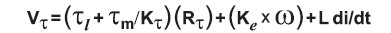Figure 5: Representing energizing/de-energizing voltage in a motor winding equation.Time Constants
Time constants are usually expressed in milli-seconds or minutes. It is the amount of time it will take to reach 63% of its final value. It will take five time constants to reach the final value. The Electrical Time Constant involves current, Mechanical Time Constant involves motor speed, and the Thermal Time constant is the rise of winding temperature.Frictional Losses
When sizing out a motor, the load torque is not the only torque the motor must overcome. Internal motor losses must be included. Below are several parameters.Coulomb Friction Torque
This is the static friction of the motor as measured with a torque watch at low speed. Usually termed ‘breakaway torque’.Viscous Damping Factor
Damping losses are proportional to speed. The losses usually include windage, eddy current, magnetic, etc. Viscous Damping
is expressed in torque/speed.Example of How to Choose the Proper Winding
Need to accelerate a load to speed within a specified time, then maintain that load at speed. The acceleration period of this move would require the most input power and will define which winding is to be used.

 Bus Voltage: 48V DC Operating Speed: 314.2 rad/s (3,000 RPM) Acceleration Torque: 0.353 Nm (50 oz-in) Running Torque: 0.177 Nm (25 oz-in)

 In this application, we will choose brushless motor EC057C-3 based on its continuous rated torque.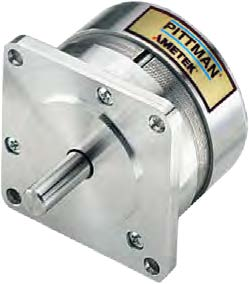The next step, choose the appropriate winding using Equation 2 below.Equation 2: Equation used to determine a winding that facilitates acceleration to load speed within a certain time.

Plugging in the values, we get:Where:= Required motor torque constant E = Supply voltage= Load torque= Motor friction torque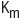= Motor constant S = line to line resistance K = Rationalization constant = 1353 for oz-in, rpm units = 9.5492 for Nm, rpm units = 1.000 for Nm, rad/s units

The number calculated here (0.115 Nm/A) is an ‘ideal’ value. It is a good idea to choose a Kt that is about 15% lower to compensate for any ‘other’ losses such as voltage drop across the drive, effects of switching inductance, etc. This results in a Kt of .098 Nm/A. From the winding choices in Table 1, the 76.4 V WDG with a Kt of .102 Nm/A would be a good choice.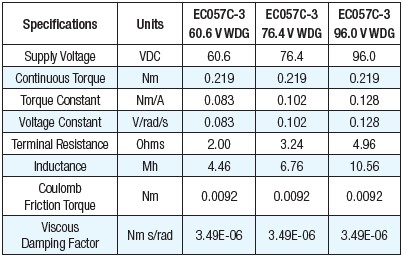It is usually unknown what the ‘other’ losses will be, but you can at least calculate the resultant voltage of the switching inductance. This can be calculated from Equation 3 below.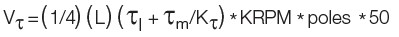Equation 3: Equation used to determine the resultant voltage of the switching inductance.

Plugging the values into Equation 3, we get

E = (1/4)(.00676)(.353+.0103/.102) * 3 * 4 * 50 = 3.61 V

Now we can use the motor equation (Equation 1.) To verify our winding choice.

E = (.353+.0103/.102)(3.24) + (.102)(314.2) + 3.61 = 47.2 V

With a supply voltage of 48 volts, the 76.4 V WDG would be an acceptable winding.Conclusion
In choosing the proper winding, you have to look beyond the torque and voltage ratings of the winding. Inductance, Coulomb Friction, and Viscous Damping all have a great effect on the winding choice. If these parameters are ignored, you run a high chance of not having enough input power to run your torque/speed profile.